Ex 3.6

Chapter 3 Class 10 Pair of Linear Equations in Two Variables
Serial order wise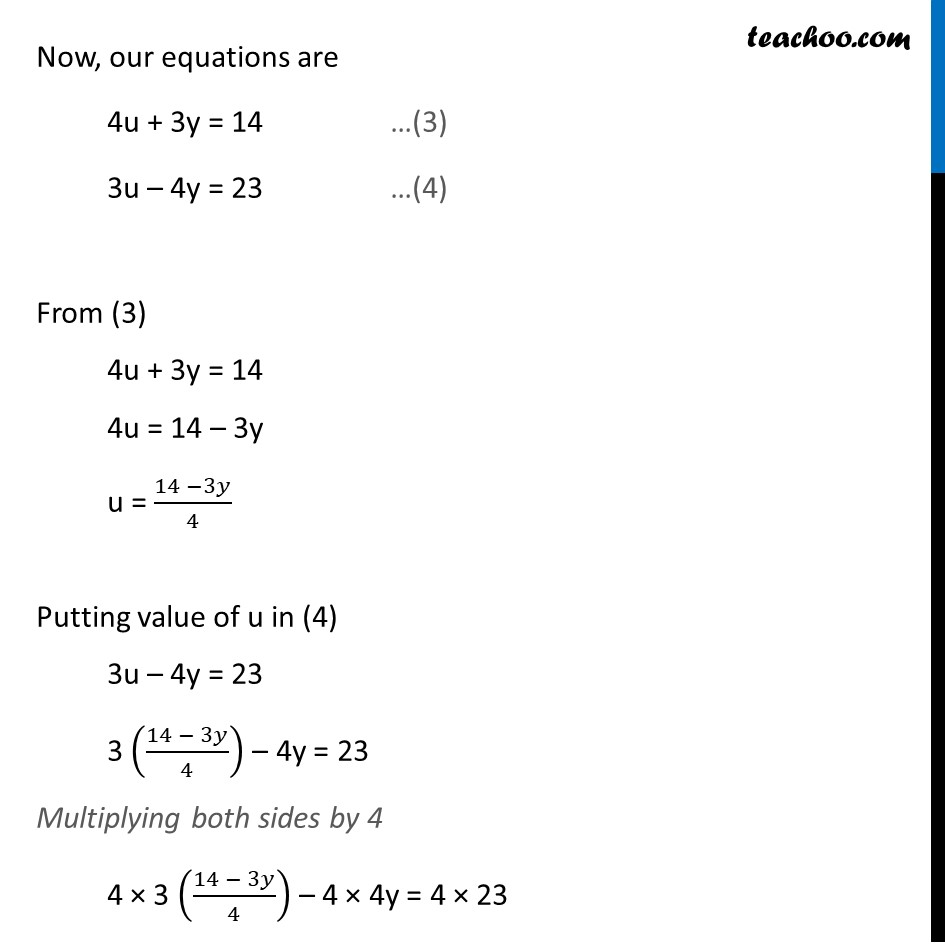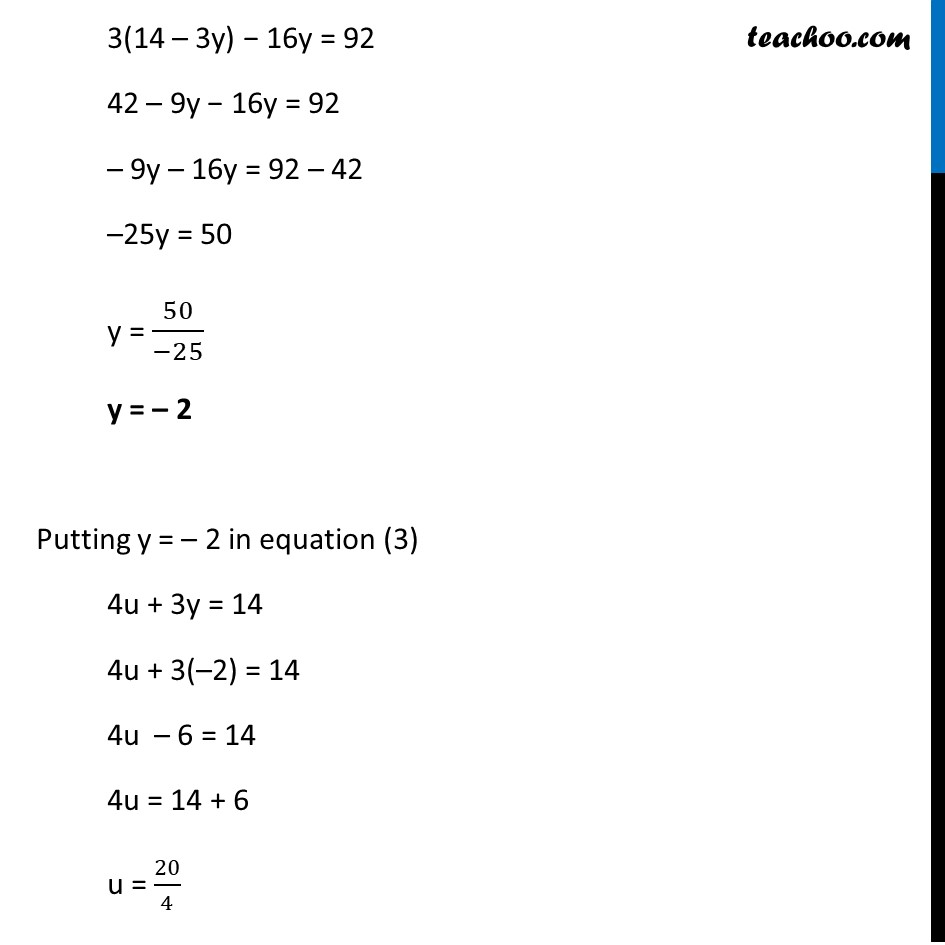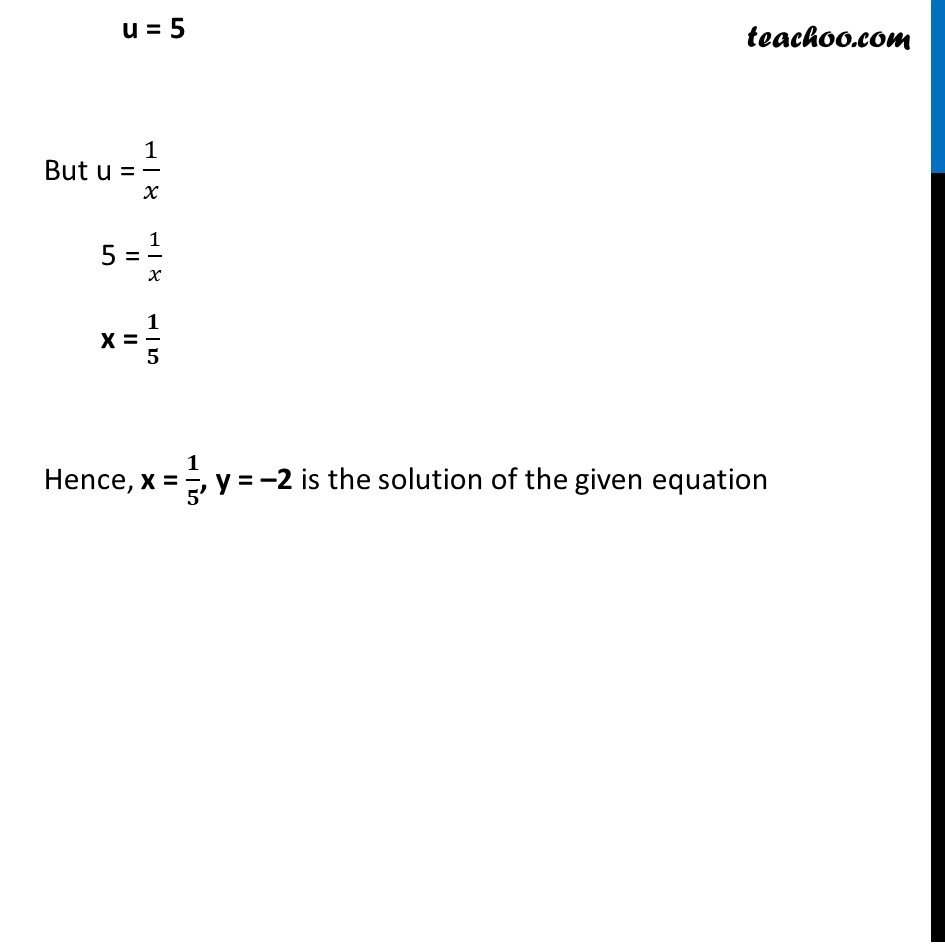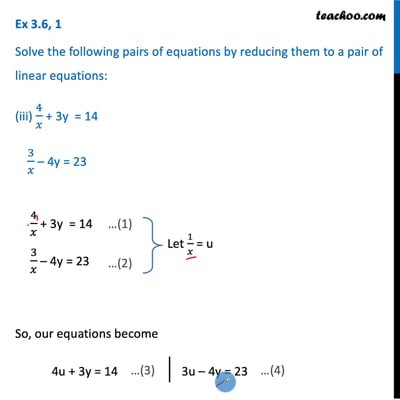This video is only available for Teachoo black users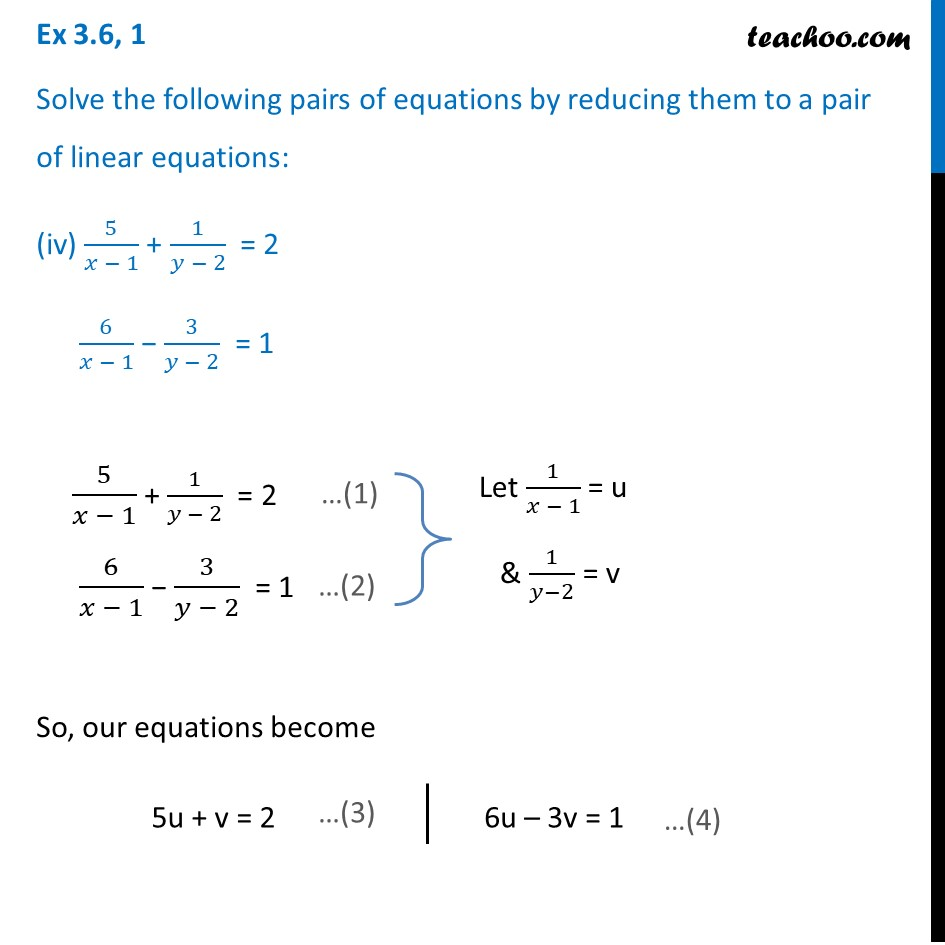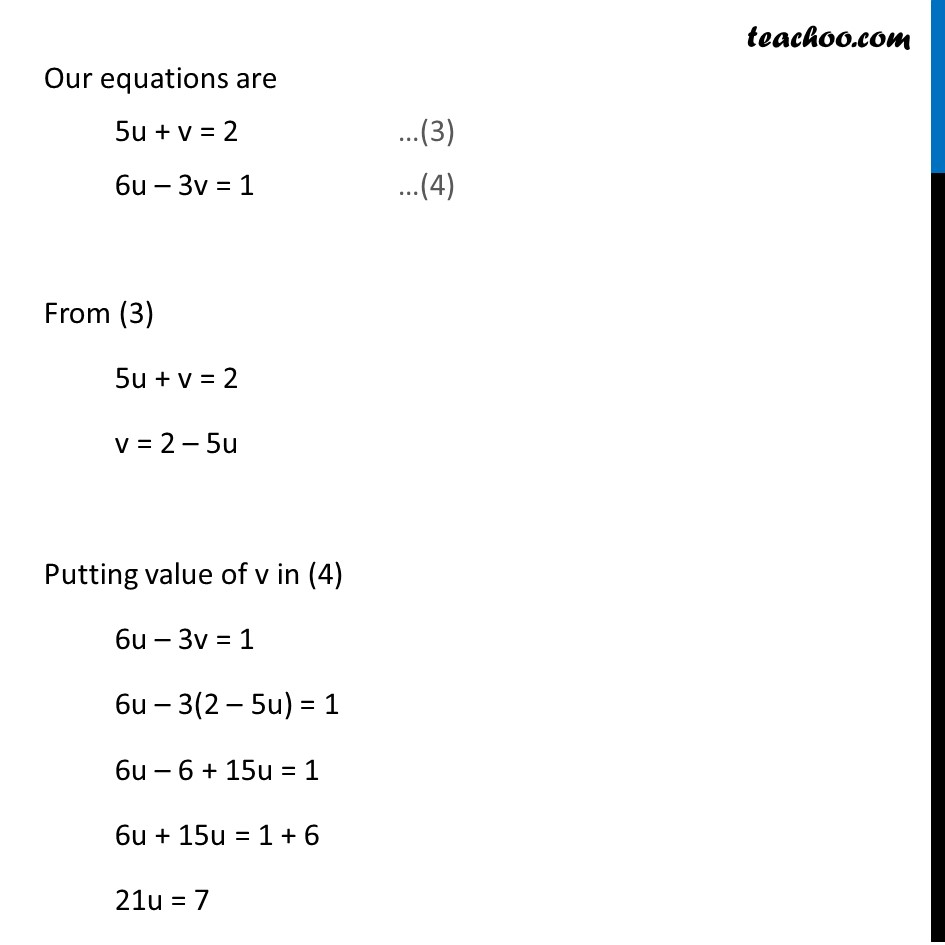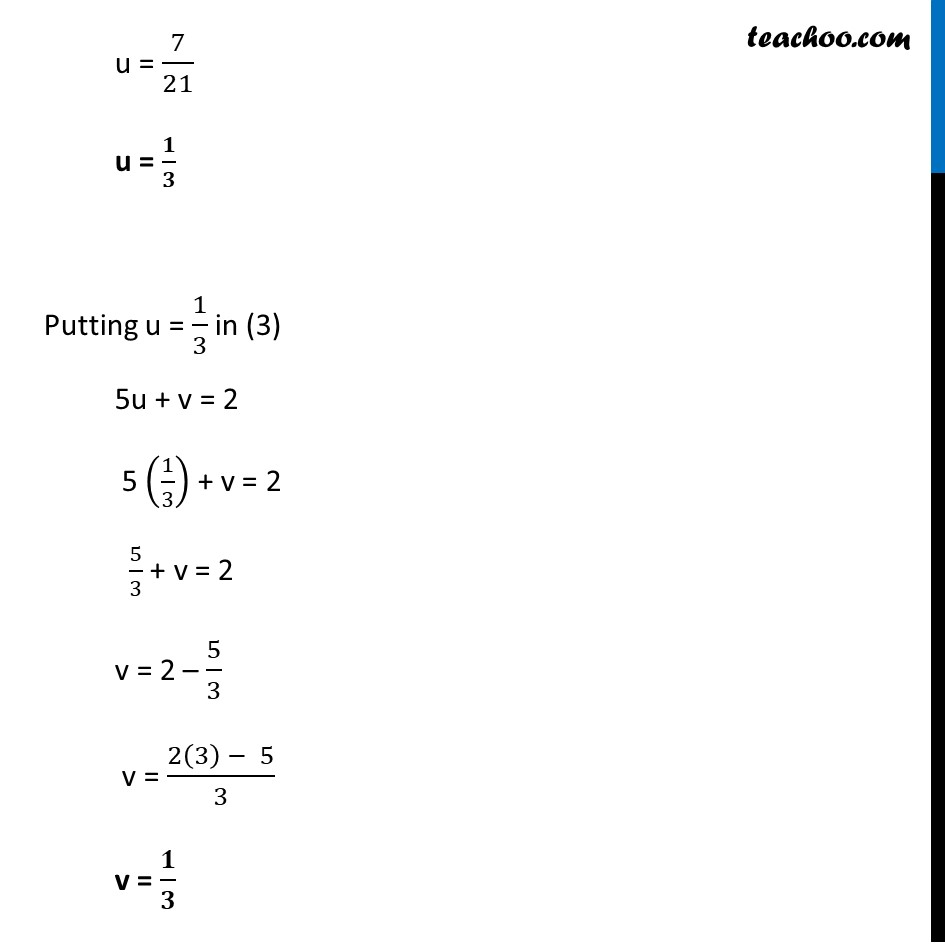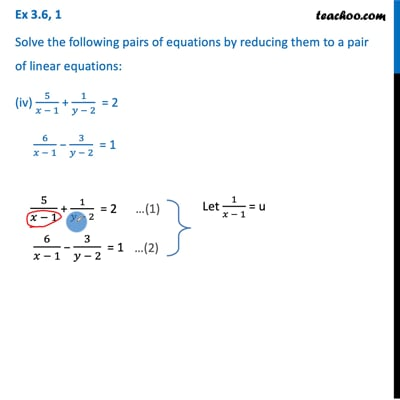This video is only available for Teachoo black users

Solve all your doubts with Teachoo Black (new monthly pack available now!)

### Transcript

Ex 3.6, 1 Solve the following pairs of equations by reducing them to a pair of linear equations: (iii) 4/𝑥 + 3y = 14 3/𝑥 – 4y = 23 4/𝑥 + 3y = 14 3/𝑥 – 4y = 23 So, our equations become 4u + 3y = 14 3u – 4y = 23 Now, our equations are 4u + 3y = 14 …(3) 3u – 4y = 23 …(4) From (3) 4u + 3y = 14 4u = 14 – 3y u = (14 −3𝑦)/4 Putting value of u in (4) 3u – 4y = 23 3 ((14 − 3𝑦)/4) – 4y = 23 Multiplying both sides by 4 4 × 3 ((14 − 3𝑦)/4) – 4 × 4y = 4 × 23 3(14 – 3y) − 16y = 92 42 – 9y − 16y = 92 – 9y – 16y = 92 – 42 –25y = 50 y = 50/(−25) y = – 2 Putting y = – 2 in equation (3) 4u + 3y = 14 4u + 3(–2) = 14 4u – 6 = 14 4u = 14 + 6 u = 20/4 u = 5 But u = 1/𝑥 5 = 1/𝑥 x = 𝟏/𝟓 Hence, x = 𝟏/𝟓, y = –2 is the solution of the given equation Ex 3.6, 1 Solve the following pairs of equations by reducing them to a pair of linear equations: (iv) 5/(𝑥 − 1) + 1/(𝑦 − 2) = 2 6/(𝑥 − 1) − 3/(𝑦 − 2) = 1 5/(𝑥 − 1) + 1/(𝑦 − 2) = 2 6/(𝑥 − 1) − 3/(𝑦 − 2) = 1 So, our equations become 5u + v = 2 6u – 3v = 1 Our equations are 5u + v = 2 …(3) 6u – 3v = 1 …(4) From (3) 5u + v = 2 v = 2 – 5u Putting value of v in (4) 6u – 3v = 1 6u – 3(2 – 5u) = 1 6u – 6 + 15u = 1 6u + 15u = 1 + 6 21u = 7 u = 7/21 u = 𝟏/𝟑 Putting u = 1/3 in (3) 5u + v = 2 5 (1/3) + v = 2 5/3 + v = 2 v = 2 – 5/3 v = (2(3) − 5)/3 v = 𝟏/𝟑 Hence, u = 1/3 & v = 1/3 We need to find x & y We know that u = 𝟏/(𝒙 − 𝟏) 1/3 = 1/(𝑥 − 1) x – 1 = 3 x = 3 + 1 x = 4 v = 𝟏/(𝒚 − 𝟐) 1/3 = 1/(𝑦 −2) y – 2 = 3 y = 3 + 2 y = 5 So, x = 4, y = 5 is the solution of our equations